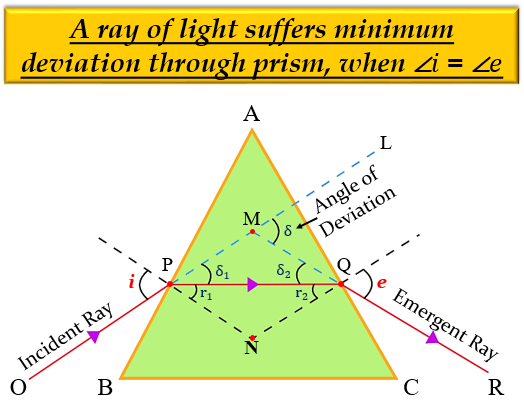# Which of the following pair of angles are equal?(a) Angle of incidence and angle of refraction.(b) Angle of prism and the angle of emergence.(c) Angle of refraction and angle of deviation.(d) Angle of incidence and angle of emergence.

The correct answer is Option (d) Angle of incidence and angle of emergence.

Explanation

The angle of incidence (i) and angle of emergence (e) will be equal when a ray of light suffers minimum deviation through a prism and the refracted ray inside the prism becomes parallel to its base, i.e. i = e.Updated on: 10-Oct-2022

105 Views